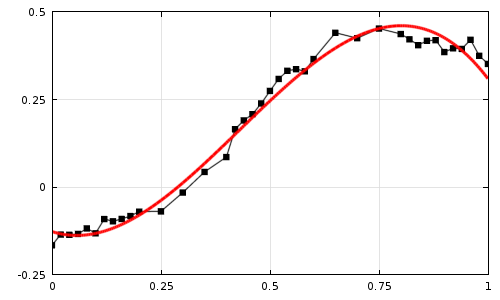## Linear and Cubic Polynomial Curve Fitting for Temperature-Cp Data

AIM

To plot the linear and cubic fit curves alongwith raw data points and to measure fitness for both the curves.

INTRODUCTION:

Curve fitting[is the process of constructing a curve , or mathematical functions, that has the best fit to a series of datapoints possibly subject to constraints.Curve fitting can involve either interpolation where an exact fit to the data is required, or smoothing  in which a "smooth" function is constructed that approximately fits the data.MATLAB PROGRAM:

clear all
close all
clc

%preparing the data
temperature=cp_data(:,1);
cp=cp_data(:,2);

%Curve fit
%Getting Coefficients of the equation using polyfit function
%Evaluating the polynomial using polyval function

coef=polyfit(temperature,cp,1);
pre_cp=polyval(coef,temperature);
coef1=polyfit(temperature,cp,3);
pre_cp1=polyval(coef1,temperature);
%plotting all the data
plot(temperature,cp,'linewidth',2.5);
hold on
plot(temperature,pre_cp,'linewidth',1.5,'color','b')
plot(temperature,pre_cp1,'linewidth',1.5,'color','c')

xlabel('Temperature[K]');
ylabel('Specific Heat[KJ/Kmol-k]');
legend('original dataset','Linear curve fit','Cubic curve fit')
grid on
%Plotting Percentage difference Curve

rmse_1 = sqrt(mean((cp-pre_cp).^2))
rmse_3 = sqrt(mean((cp-pre_cp1).^2))


PROGRAM DESCRIPTION:

The data contains the temperature and Cp data is called which is aset of rows and columns and a curve is plotted based on the values.Then inorder to plot a curve of best fit the coefficients of the required curve is calculated using the polyfit function and the equation is evaluated and the  cp values are predicted using the polyval function.Plotting the linear and cubic curves and finally the root mean square errors are calculated using the equation.where, pre_Cp=predicted values of Cp

Cp=Initial values of Cp

RESULTS:RMSE values:Program for curvefit of a Linear  1st Degree Polynomial using split method:

clear all
close all
clc

%preparing the data
temperature=cp_data(:,1)
cp=cp_data(:,2);

%Curve fit
%Getting Coefficients of the equation using polyfit function
%Evaluating the polynomial using polyval function

coef=polyfit(temperature,cp,1);
pre_cp=polyval(coef,temperature);
%splitting the curve
a=1;
b=320;
for i=1:10
coef(i,:)=polyfit(temperature(a:b),cp(a:b),1);
pre_cp(a:b)=polyval( coef(i,:),temperature(a:b));
a=b+1;
b=b+320;
end

%plotting all the data
plot(temperature,cp,'linewidth',2.5);
hold on
plot(temperature,pre_cp,'linewidth',1.5,'color','b')

xlabel('Temperature[K]');
ylabel('Specific Heat[KJ/Kmol-k]');
legend('original dataset','Linear curve fit','Cubic curve fit')
grid on
%Plotting Percentage difference Curve

rmse_1 = sqrt(mean((cp-pre_cp).^2))


Output:Program for curvefit of a Cubic 3rd Degree Polynomial using split method:

clear all
close all
clc

%preparing the data
temperature=cp_data(:,1)
cp=cp_data(:,2);

%Curve fit
%Getting Coefficients of the equation using polyfit function
%Evaluating the polynomial using polyval function

coef=polyfit(temperature,cp,3);
pre_cp1=polyval(coef,temperature);
%splitting the curve
a=1;
b=320;
for i=1:10
coef1(i,:)=polyfit(temperature(a:b),cp(a:b),3);
pre_cp1(a:b)=polyval( coef1(i,:),temperature(a:b));
a=b+1;
b=b+320;
end

%plotting all the data
plot(temperature,cp,'linewidth',2.5);
hold on
plot(temperature,pre_cp1,'linewidth',1.5,'color','b')

xlabel('Temperature[K]');
ylabel('Specific Heat[KJ/Kmol-k]');
legend('original dataset','Linear curve fit','Cubic curve fit')
grid on
%Plotting Percentage difference Curve

rmse_1 = sqrt(mean((cp-pre_cp1).^2))


Ouptput:Errors Encountered:This error occured while plotting the third degree polynomial.It was fixed using the curve fit application.Results using the curve fit application:Code generated using Curvefit application:

function [fitresult, gof] = createFit1(temperature, cp)
%CREATEFIT1(TEMPERATURE,CP)
%  Create a fit.
%
%  Data for 'untitled fit 1' fit:
%      X Input : temperature
%      Y Output: cp
%  Output:
%      fitresult : a fit object representing the fit.
%      gof : structure with goodness-of fit info.
%

%  Auto-generated by MATLAB on 13-Feb-2020 22:53:32

%% Fit: 'untitled fit 1'.
[xData, yData] = prepareCurveData( temperature, cp );

% Set up fittype and options.
ft = fittype( 'poly3' );
opts = fitoptions( 'Method', 'LinearLeastSquares' );
opts.Normalize = 'on';
opts.Robust = 'Bisquare';

% Fit model to data.
[fitresult, gof] = fit( xData, yData, ft, opts );

% Plot fit with data.
figure( 'Name', 'untitled fit 1' );
h = plot( fitresult, xData, yData );
legend( h, 'cp vs. temperature', 'untitled fit 1', 'Location', 'NorthEast', 'Interpreter', 'none' );
% Label axes
xlabel( 'temperature', 'Interpreter', 'none' );
ylabel( 'cp', 'Interpreter', 'none' );
grid on



Resulting plot using Curve Fit application:References:

Mathworks

### Stalagmite Function Optimization Using Matlab Alan V Abraham · 2020-02-18 09:14:44

AIM: To optimise the stalagmite function and to find the global maxima of the function. Introduction: A genetic algorithm is a optimization technique inspired by Charles Darwin's theory of natural evolution. This algorithm reflects the p Read more

### Program to Simulate the Transient Behaviour of a Simple Pedulum and to Create Animation of its Motion Alan V Abraham · 2020-02-12 11:56:01

AIM: To simulate the transient behaviour of a simple pendulum and to create an animation of its motion. INTRODUCTION: A pendulum is a mass on an arm. The arm is attached to a pivot, which is a point to swing from.The mass will naturally hangs down due to gr Read more

### Matlab Program To Output PV Diagram And Efficiency Of Ottocycle. Alan V Abraham · 2020-02-11 15:48:58

Aim To create a PV diagram and to output the Efficiency Introduction An Ottocycle is a thermodynamic cycle that describes the changes in pressure,volume, temperature and heat.It is the thermodynamic cycle most commonly found in automobile engines.It consists of two i Read more

### Simulation Of Forward Kianematics Of A 2R Robotic Arm Alan V Abraham · 2020-02-11 06:13:38

Aim:  To simulate the forward kinematics of a 2R Robotic arm   Introduction:   With the day by day advancement in technology, robotics and automation had a great impact in the industry.The Robotic arm is used for various multiple industrial applicatio Read more